# CART: Classification & Regression Trees

## Metrics

### Gini impurity

• Measure of how often a randomly chosen element from the set would be incorrectly labeled if it was labeled randomly according to the distribution of the labels in the subset.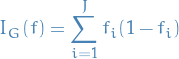1

#### Procedure

1. Draw random sample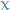from the set (which in reality has label, say,)
2. Classifyaccording to the distribution of the labels
3. Compute probability of misclassification, i.e. proportion of elements not labeled4. Compute Gini coefficient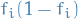, where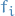is the proportion of labelin the set
5. Perform step 1-4 for the child nodes (nodes after making some split)
6. Compare sum of coefficient for child nodes with parent node
7. Perform steps 1-6 for different features / independent variables / attributes to find the one with the best (i.e. smallest) coefficient and go ham (i.e. make a split).

### Information gain

• In information theory and machine learning: information gain is a synonym for the Kullback-Leibner divergence
• In context of decision trees: sometimes used synonymously with mutual information, which is the expected value of the KL-divergence of the univariate probability distribution of one variable from the conditional distribution of this variable given the other one.

#### Notation

•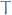is the set of training examples
•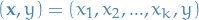is a training example
•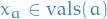is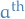attribute
•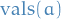is all possible value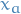can take

#### Definition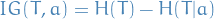or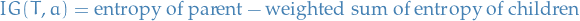or best: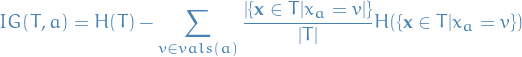2

which is just saying that Information Gain is the difference between the entropy for the entire training set and the weighted (by portion of samples which will fall into this node) sum of the entropy in the child-nodes.

Remember, Information Gain only makes sense when it comes to classification, not regression.

• Difference between the entropy of a nodeand the sum of entropy of the children given that we decide to split on attribute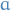• We take the difference between uncertainty before and after the split, which gives us a measure of how much more certain we are by performing said split.
• How much uncertainty we remove by making the split on this independent variable

#### Why the entropy?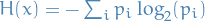•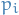is the probability of class• Entropy is simply a function chosen to satisfy some criterias that one would intuitively want from a uncertainty-measure:
1.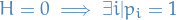2.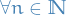,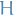is maximum when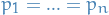, i.e. we are most uncertain when every possiblity is equally likely.
3.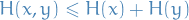, with equality iffand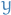are independent
4.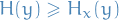, i.e. uncertainty regardingis never increased by knowing5. Any change towards equalization of the probabilities increases. Greater uncertainty ⇒ greater entropy.

#### Example

Consider an example data-set of 14 samples, with attributes:

•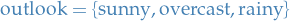•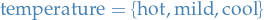•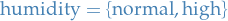•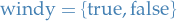And our (binary) dependent variable / output is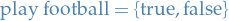.

Let's assume we make the first split on windy (in reality we would consider splitting on each of the independent variables and then use the one with the best metric), where the resulting tree has two nodes.

### Variance reduction

• Often used when target is continuous (regression tree)
• Variance reduction of a node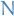is the total reduction of the variance of the target variabledue to the split at this node
• target variablebeing the value of the sample
• look for the attribute / independent variable with the split "explaining" the most variance of our target variable, i.e. has the variance reduced the most

#### Defintion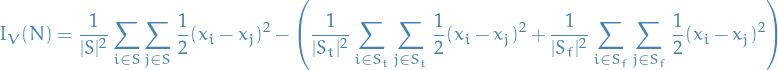3

where

•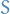- the set of presplit sample indices
•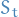- set of sample indices for which the split test is true
•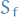- set of sample indices for which the split test is false

Each of the above summands are indeed variance estimates, though, written in a form without directly referring to the mean.